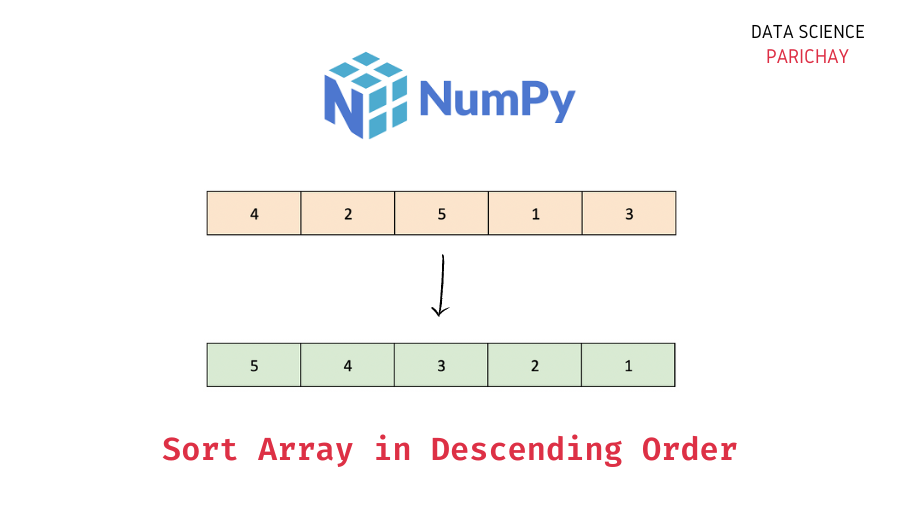# Sort Numpy Array in Descending Order

The Numpy library in Python comes with a number of useful functions and techniques to work with and manipulate arrays. In this tutorial, we will look at how to sort a Numpy array in descending order with the help of some examples.

## Is there a direct function to sort a Numpy array in descending order?

No. The `numpy.sort()` function sorts a Numpy array in ascending order. It also does not take any parameters or configurations to sort the array in descending order. However, there’s a workaround.

To sort a Numpy array in descending order, first, sort it in ascending order and then reverse the array using slicing. The following is the syntax –

```# sort numpy array ar in descending order
np.sort(ar)[::-1]```

## Steps to sort a Numpy array in descending order

Let’s take a look at a step-by-step example of using the above syntax –

### Step 1 – Create a Numpy array

First, we will create a one-dimensional Numpy array that we will be using throughout this tutorial.

```import numpy as np

# create numpy array
ar = np.array([4, 1, 6, 3, 7, 5, 2])
# display the array
print(ar)```

Output:

`[4 1 6 3 7 5 2]`

Here, we used the `numpy.array()` function to create a Numpy array containing some numbers. The length of the array is 7.

### Step 2 – Sort the Numpy array (in ascending order)

Use the `numpy.sort()` function to sort the array created above in ascending order (As already discussed, you cannot use this function to directly sort an array in descending order). It returns a sorted copy of the original array.

📚 Data Science Programs By Skill Level

Introductory

Intermediate ⭐⭐⭐

🔎 Find Data Science Programs 👨‍💻 111,889 already enrolled

Disclaimer: Data Science Parichay is reader supported. When you purchase a course through a link on this site, we may earn a small commission at no additional cost to you. Earned commissions help support this website and its team of writers.

```# sort the array
sorted_ar = np.sort(ar)
# display the sorted array
print(sorted_ar)```

Output:

`[1 2 3 4 5 6 7]`

The resulting array is sorted in ascending order.

Note – You can also use the instance method `numpy.ndarray.sort()` to sort a Numpy array in ascending order. This function modifies the array in-place.

### Step 3 – Reverse the array using slicing

Now that we have the array sorted in ascending order, we only need to reverse it to get the descending order array.

To reverse the array, slice the entire array (from start to end) with a step of -1. This will return the reversed array.

```# reverse the sorted array
print(sorted_ar[::-1])```

Output:

`[7 6 5 4 3 2 1]`

You can see that the array elements are now sorted in descending order.

If you’re confused about the slicing operation, look at its syntax `ar[start:end:step]`. Here, since we want to slice the entire array, we do not specify explicitly start and end indices.

The `step` value in slicing indicates the step size. Using -1 as the step size will return the array resulting from traversing the sliced part in the opposite direction (from right to left) 1 element at a time. Thus, using the slice operation `ar[::-1]` will reverse the array.

We can combine the code from step 2 and step 3 in a single line and avoid using the extra variable.

```# sort array in descending order
print(np.sort(ar)[::-1])```

Output:

`[7 6 5 4 3 2 1]`

We get the same result as above.

## Summary – Sort Numpy array in descending order

In this tutorial, we looked at how to sort a Numpy array in descending order. The following is a short summary of the steps involved –

1. Create a Numpy array (skip this step if you already have Numpy array to operate on).
2. Sort the array in ascending order using the `numpy.sort()` function.
3. Reverse the array using slicing (`ar[::-1]`) to get the array with elements in descending order.

You might also be interested in –

•